## Tuesday 7 March 2017

### 7 Habits of highly effective C# Programmer(3 Series) - Part 2

3. Use `Yield` Keyword in Statement
This is one of the keyword that should be widely used when you know the input’s length is un-deterministic. It can be used to create composable API’s using generics. Yield follows nothing but a deferred execution model.
Let us look into the example before any explanation, with an usecase. Until you  understand the use case where it can be used, it is tough to catch up the logic and usage behind the Yield keyword.
private static IEnumerable<int> TestFibbonacciWithoutYield(int limit)
{
int f1 = 0, f2 = 1, f3 = 0;
Console.WriteLine("Fibonacci Series:");
//IList collections is needed to hold values
IList<int> collections = new List<int>();

for (int i = 0; i < limit; i++)
{
f3 = f1 + f2;
Console.WriteLine("Fibonacci Series sequence:" + i);
f1 = f2;
f2 = f3;
}
return collections;
}

In the above function we have iterated through limit to print the fibbononacci series, when the function is called with
IEnumerable<int> collections = Program.TestFibbonacciWithoutYield(10);

foreach (int fibonnacciSeries in Program.TestFibbonacciWithoutYield(10))
{
Console.WriteLine(fibonnacciSeries + " ,");
}

The output will be as below.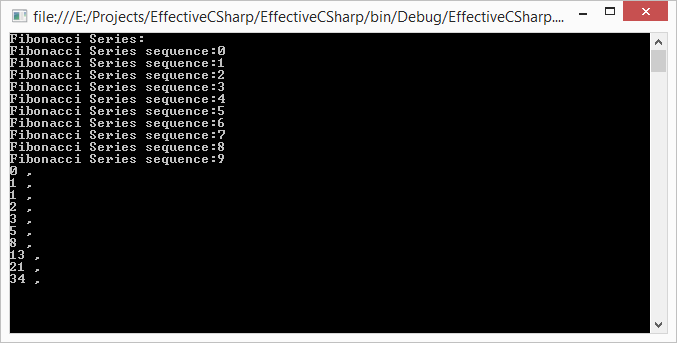The collection is filled with values, then the collection is printed, what if the limit that is set as  TestFibbonacciWithoutYield(1 million) (yes with 6 zeroes !!!) is million so a list will be created with one million integer values are traversed and printed.
So the solution is to use Yield keyword to remove the unnecessary list that needs to hold the values   which in turn  holds the application memory.

Below code does use the yield keyword
private static IEnumerable<int> TestFibbonacciWithYield(int limit)
{
int f1 = 0, f2 = 1, f3 = 0;
Console.WriteLine("Fibonacci Series:");
//---You dont need the IList collections;
for (int i = 0; i < limit; i++)
{
f3 = f1 + f2;
Console.WriteLine("Fibonacci Series sequence(i): "  + i);
f1 = f2;
f2 = f3;
yield return f1;

}
}
Note in the above example even though the return type of TestFibbonacciWithYield() is IEnumerable<int> , there are no collections that are created in the method.
When we call the method as below.
static void Main(string[] args)
{
IEnumerable<int> collections = Program.TestFibbonacciWithYield(10);

foreach (int fibonnacciSeries in collections)
{
Console.WriteLine(fibonnacciSeries + " ,");
}
}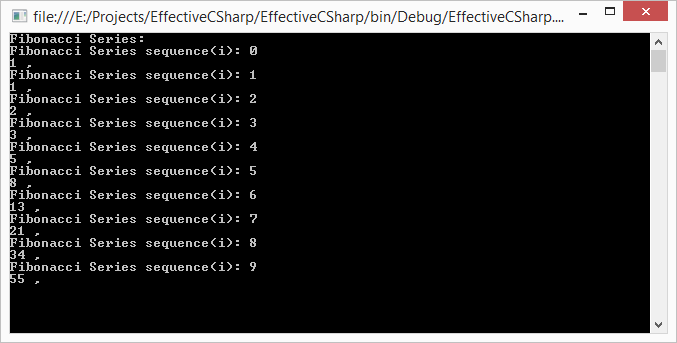The output denotes the fibonnaci series is generated only when the foreach has accessed the element instead of running all once.
So what happens here exactly in Yield Keyword?
TestFibbonacciWithoutYield()  method, allocates the entire storage for the sequence of elements, and TestFibbonacciWithYield(), ask for the next element on the input sequence only when needed, and they produce the next value on the output sequence only when the calling code asks for it.
Yield plays an interesting trick, it returns a value and retains information about its current location and current state of its internal iteration. Both the input and output are iterators. This is called as continuation method. Continuation methods keep track of their state and resume execution at their current location when code enters them again.
So what is advantage other than reduced need to create a new collection in the TestFibbonacciWithoutYield()  method ?
Yield provides deferred lazy loading meaning, you can access elements whenever you need it. Imagine a scenario where the input limit for the Fibonacci series is million (Yes 1 with six zeroes), then we might need to hold a list that has 1 million records in the memory. You might not want that, because all you need is to print them.
IEnumerable<int> collections = Program.TestFibbonacciWithYield(100000).Skip(50000);
In above command will do a deferred loading to skip first 50,000 records and print the remaining values. Imagine the memory you have said. You can use Yield in places where the input is un-deterministic and you need to make sure the application handles the deferred loading. I wish Yield is available in javascript(ECMA 5.0 or earlier, I heard it is in ECMA 6).

4. Understanding Closure
Due to all the invasion of functional programming concept into the .Net 3.0 and forward, we are getting many benefits in oops language like C# , functional way of thinking. In functional programming world the functions are passed from one functions to another as we pass objects from one object to another in OOPS World. Closure is very important concept that needs to be understood, it is fairly simple and straightforward. I like to keep the sample as simple as possible. I have dumbed down the example, so you can understand the concepts of closure first and then we will see why you should bother understanding them in the first place.
Before starting our understanding, we need to understand a term called “First- Class Function”.
First class function is nothing but a variable that can hold functions.
i.e... function that can be passed as a variable and stored in a  data-structure. As of now, if you know what is function pointer then you can consider it as First class function. In C# we can achieve it by lambda functions or anonymous methods. Explaining more about it and talking about functional programming is out of scope of our blog. So we will dive into an example straightaway.

private static int  StraightForwardFunction(int noToPrint)
{

return IncrementingFunction(noToPrint);
}
private static int IncrementingFunction(int value)
{
value = value + 1;
return value;
}
In above StraightForwardFunction() function we are passing the noToPrint to Incrementing function and the value is incremented and passed back.
static void Main(string[] args)
{
Console.WriteLine(ClosureProgram.StraightForwardFunction(5));
Console.WriteLine(ClosureProgram.StraightForwardFunction(6));
}
When we run the StraightForwardFunction, the output will be as below with incremented value 6 and 7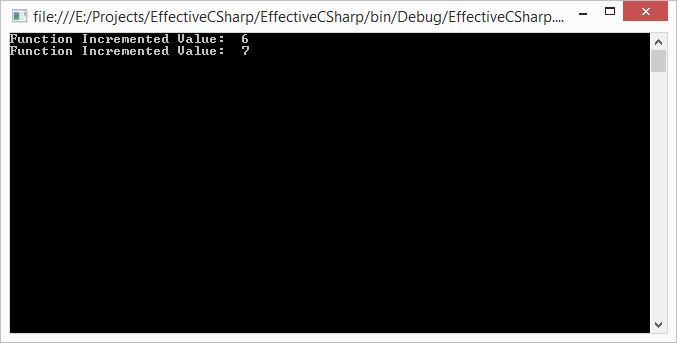Let us see how delegate function works in this regard.
public static Func<int, int> TestClosure()
{
var mainScope = 0;
return delegate (int local)
{
mainScope = mainScope + 1;
return local + mainScope;
};

}
In the above function TestClosure(), when called twice with value as below
var closureCall = TestClosure();
Console.WriteLine(\$"Closure Incremented Value:  {closureCall(5)}");
Console.WriteLine(\$"Closure Incremented Value:  {closureCall(6)}");
We should expect the value to be incremented to 6 and 8 respectively. But the answer is not 6 and 7. See below.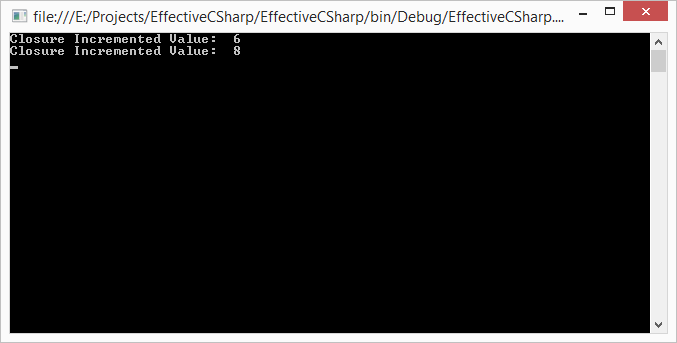When the function is called twice, the value is persisted with the delegate that compose of incrementing the value and the value is incremented with last main scope variable.
Strange right? All the years what we have read about the scopes enclosed in curly braces seems to be wrong with the above delegate. Let us see what happens when a delegate used to do some work when the IL is created.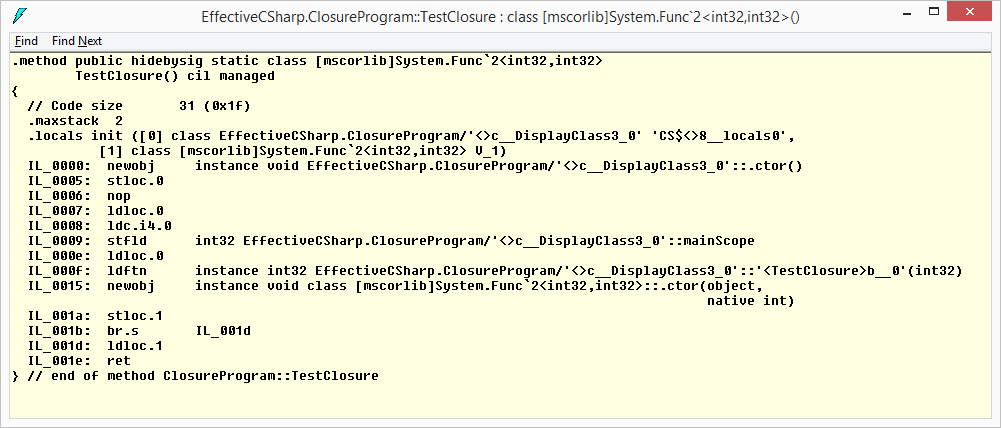The closure function is enclosed with class and so the variables are persisted when we use the delegate, which is the reason, why the variable incremented to 8 instead of 7.
But in case of StraightForwardFunction, the IL created was only as function as below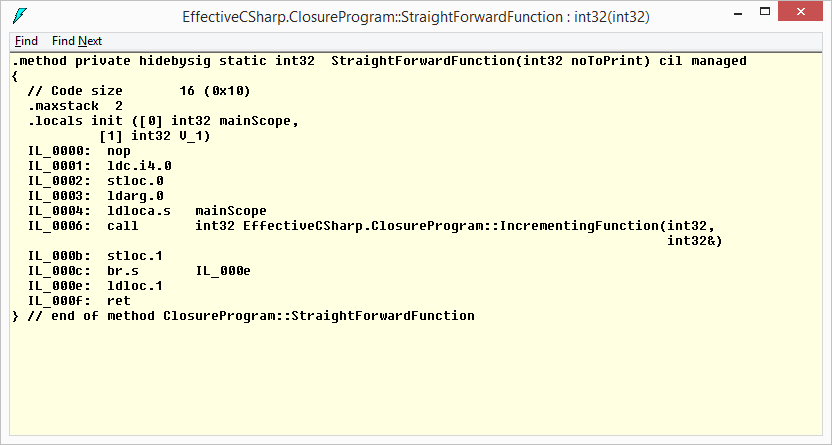But why should I care what IL and C# compiler does ?, how does knowing this make me effective c# programmer. The answer is, IT DOES.
As developers we’ve grown accustomed to looking at the lifetime of local variables in a very simple way. Variables come into scope when we declare them, and they are garbage collected when the corresponding block closes.
Closures and captured variables change those rules, When you use a variable that is bound by closure, it’s reference to the variable does not go out of scope until the last delegate referencing that captured variable goes out of scope. Under some circumstances it may even last longer.
What happens when we hold an expensive resources that has implemented IDisposable and needs to be explicitly cleaned up? So you need to understand how objects created are returned from methods and you must ensure that the closure clean them up for you.